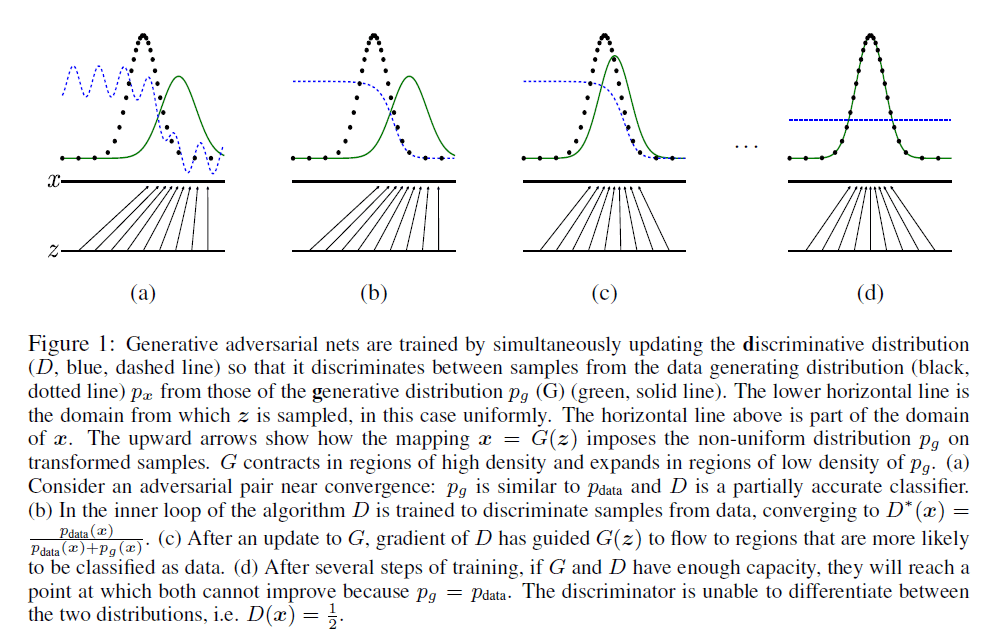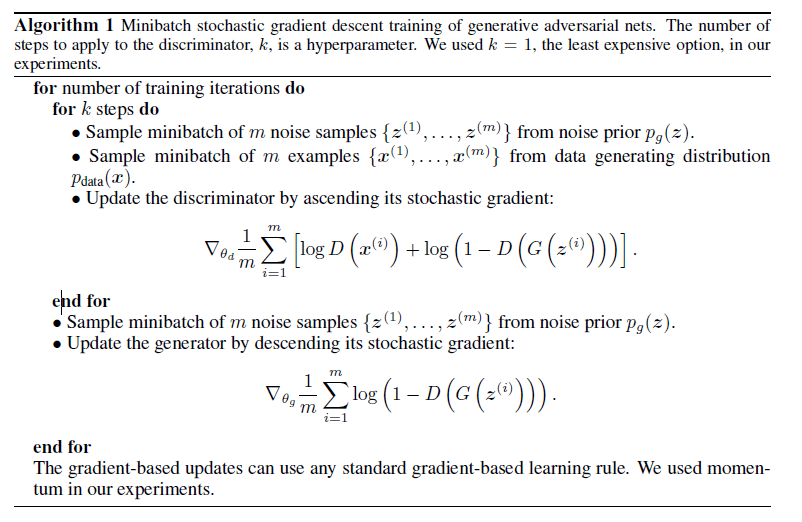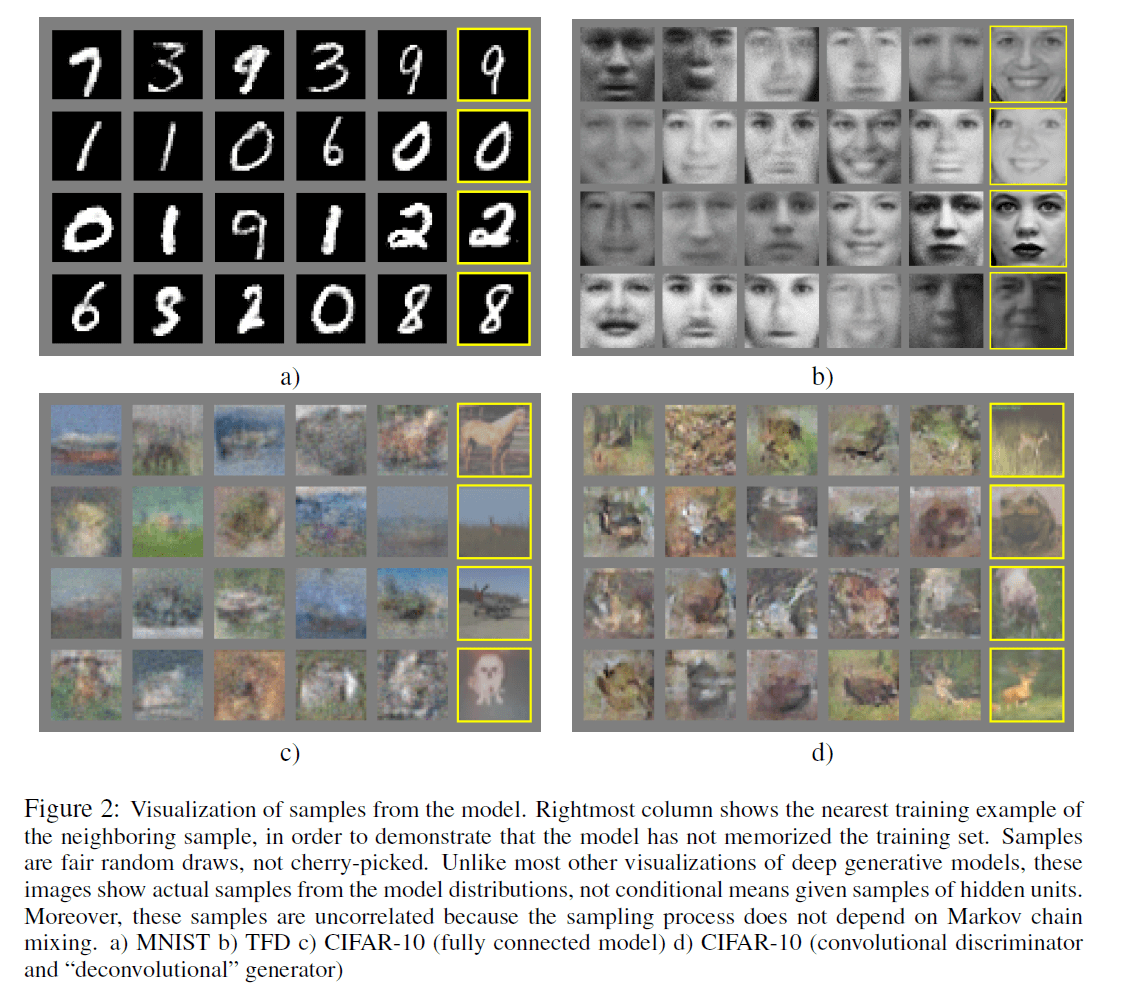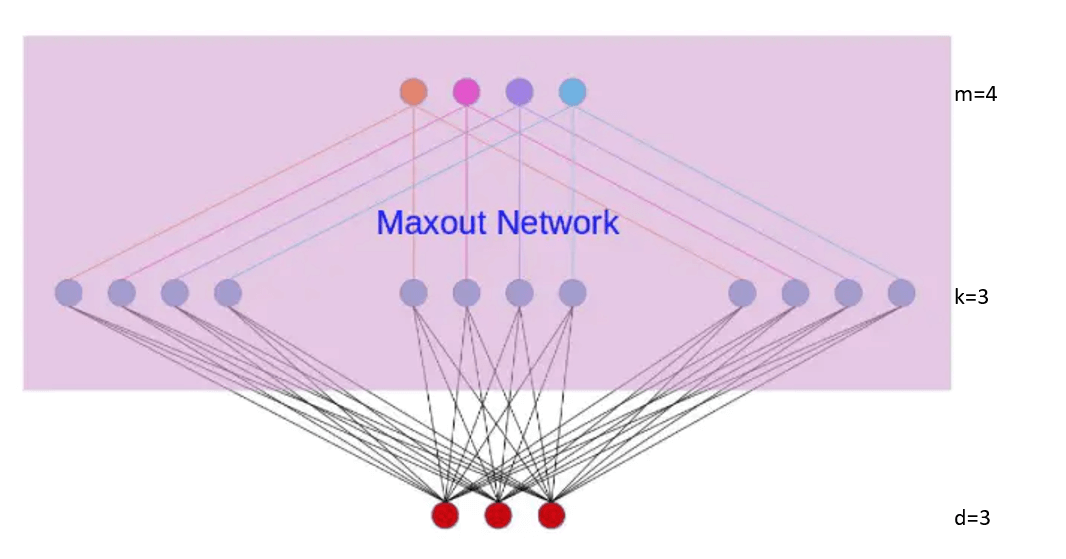## GAN，maxout激活函数，KL散度，JS散度

Posted by x-jeff on October 30, 2021

# 2.Related work

$\min \limits_{G} \ \max \limits_{D} V(D,G) = \mathbb{E}_{\mathbf x \sim p_{data}(\mathbf x)} [\log D(\mathbf x)]+\mathbb{E}_{\mathbf z \sim p_{\mathbf z} (\mathbf z)} [\log (1-D(G(\mathbf z)))] \tag{1}$# 4.Theoretical Results

## 4.1.Global Optimality of $p_g = p_{data}$

【命题1（proposition 1）】给定G，最优的D为：

$D^*_G(\mathbf x)=\frac{p_{data}(\mathbf x)}{p_{data}(\mathbf x) + p_g(\mathbf x)} \tag{2}$

【证明】模型D的训练准则为：给定模型G，最大化下式：

\begin{align} V(G,D) &= \int_{\mathbf x} p_{data} (\mathbf x) \log( D(\mathbf x)) dx + \int_{\mathbf z} p_{\mathbf z} (\mathbf z) \log (1-D(g(\mathbf z)))dz \\&= \int_{\mathbf x} p_{data}(\mathbf x) \log (D(\mathbf x))+p_g(\mathbf x) \log(1-D(\mathbf x))dx \tag{3} \end{align}

\begin{align} C(G) &= \max \limits_{D} V(G,D) \\&= \mathbb{E}_{\mathbf x \sim p_{data}} [\log D^*_G(\mathbf x)] + \mathbb{E}_{\mathbf z \sim p_{\mathbf z}} [\log (1-D^*_G(G(\mathbf z)))] \\&= \mathbb{E}_{\mathbf x \sim p_{data}}[\log D^*_G(\mathbf x)] + \mathbb{E}_{\mathbf x \sim p_g} [ \log(1-D^*_G(\mathbf x))] \\&= \mathbb{E}_{\mathbf x \sim p_{data}} \left[ \log \frac{p_{data}(\mathbf x)}{p_{data}(\mathbf x)+p_g (\mathbf x)} \right] + \mathbb{E}_{\mathbf x \sim p_g} \left[ \log \frac{p_g(\mathbf x)}{p_{data}(\mathbf x)+p_g(\mathbf x)} \right] \end{align}\tag{4}

【定理1（Theorem 1）】当且仅当$p_g=p_{data}$时，$C(G)$取到全局最小值，为$-\log 4$。

【证明】假设存在某一模型G，使得$p_g = p_{data}$，此时有$D^*_G(\mathbf x)=\frac{1}{2}$。代入式(4)，便可得到$C(G)=\log \frac{1}{2}+\log \frac{1}{2}=-\log 4$：

$\mathbb{E}_{\mathbf x \sim p_{data}} [-\log 2]+\mathbb{E}_{\mathbf x \sim p_g} [-\log 2]=-\log 4$

$C(G)=\int_{\mathbf x} p_{data} (\mathbf x) \log (\frac{p_{data}(\mathbf x)}{p_G(\mathbf x)+p_{data}(\mathbf x)})+p_G(\mathbf x)\log (\frac{p_G(\mathbf x)}{p_G(\mathbf x)+p_{data}(\mathbf x)}) dx \tag{4.1}$

$C(G)=\int_{\mathbf x}(\log 2-\log2)p_{data}(\mathbf x)+p_{data} (\mathbf x) \log (\frac{p_{data}(\mathbf x)}{p_G(\mathbf x)+p_{data}(\mathbf x)})+(\log 2-\log 2)p_G(\mathbf x)+p_G(\mathbf x)\log (\frac{p_G(\mathbf x)}{p_G(\mathbf x)+p_{data}(\mathbf x)}) dx \tag{4.2}$

$C(G)=-\log 2\int_{\mathbf x} p_G(\mathbf x)+p_{data}(\mathbf x)dx+\int_{\mathbf x} p_{data}(\mathbf x) (\log 2+ \log (\frac{p_{data}(\mathbf x)}{p_G(\mathbf x)+p_{data}(\mathbf x)}))+p_G(\mathbf x)(\log 2+\log(\frac{p_G(\mathbf x)}{p_G(\mathbf x)+p_{data}(\mathbf x)}))dx \tag{4.3}$

$-\log 2\int_{\mathbf x} p_G(\mathbf x)+p_{data}(\mathbf x)dx=-\log 2(1+1)=-2\log 2=-\log 4 \tag{4.4}$

$\log 2+\log(\frac{p_{data}(\mathbf x)}{p_G(\mathbf x)+p_{data}(\mathbf x)})=\log(2 \frac{p_{data}(\mathbf x)}{p_G(\mathbf x)+p_{data}(\mathbf x)})=\log(\frac{p_{data}(\mathbf x)}{(p_G(\mathbf x)+p_{data}(\mathbf x))/2}) \tag{4.5}$

$C(G)=-\log 4+\int_{\mathbf x}p_{data}(\mathbf x) \log(\frac{p_{data}(\mathbf x)}{(p_G(\mathbf x)+p_{data}(\mathbf x))/2}) dx + \int_{\mathbf x}p_G(\mathbf x) \log(\frac{p_G(\mathbf x)}{(p_G(\mathbf x)+p_{data}(\mathbf x))/2}) dx \tag{4.6}$

$C(G) = -\log(4) + KL(p_{data} \parallel \frac{p_{data}+p_g}{2}) + KL(p_g \parallel \frac{p_{data}+p_g}{2})\tag{5}$

$C(G) = -\log(4)+2\cdot JSD(p_{data} \parallel p_g) \tag{6}$

# 5.ExperimentsGAN的一个缺点就是在训练过程中，模型D和模型G的更新要很好的同步。首先，模型D如果训练的过好（相比于同期的模型G来说），那么会导致训练模型G时梯度消失，模型G的loss降不下去。而如果模型D训练的过差（相比于同期的模型G来说），又会导致模型G的梯度不准。所以模型D必须得训练的不好不坏才行（即和同期的模型G很好的同步）。此外，模型G也不应该被训练的过好（相比于同期的模型D），这会使得模型G生成图像趋向于和训练数据一模一样，就像直接copy训练数据一样，这显然也不是我们希望的（我们希望的是生成图像和$p_{data}$的分布一样，其具有一定的自创性，并不是完全的一样）。这也是GAN训练难问题的原因所在。

GAN的优点是不再需要马尔科夫链和近似推理。

# 8.maxout激活函数maxout network可以看做是在两个相邻隐藏层之间又加入了一个激活函数层。隐藏层节点的输出表达式为：

$h_i(x)=\max \limits_{j \in [1,k]} \ z_{ij}$ $where \ z_{ij}=x^TW_{...ij}+b_{ij}, \ and\ W\in \mathbb{R}^{d\times m \times k}$

W是三维的。d为上一层的神经元数目（本例中d=3）；m为相邻的下一层的神经元数目（本例中m=4），k为激活函数层的节点数（本例中k=3）。maxout激活函数是非线性的。

# 9.KL散度

## 9.1.定义

$KL(P \parallel Q)=\sum P(x) \log \frac{P(x)}{Q(x)}$ $KL(P \parallel Q)=\int P(x) \log \frac{P(x)}{Q(x)} dx$

## 9.2.计算实例

$A(0)=\frac{1}{2},A(1)=\frac{1}{2}$ $B(0)=\frac{1}{4},B(1)=\frac{3}{4}$ $C(0)=\frac{1}{8},C(1)=\frac{7}{8}$

$KL(A \parallel B)=\frac{1}{2} \log (\frac{1/2}{1/4}) + \frac{1}{2} \log (\frac{1/2}{3/4})=\frac{1}{2}\log (\frac{4}{3})$ $KL(A \parallel C)=\frac{1}{2} \log (\frac{1/2}{1/8}) + \frac{1}{2} \log (\frac{1/2}{7/8})=\frac{1}{2}\log (\frac{16}{7})$

## 9.3.性质

【非负性】相对熵恒为非负：$KL(P \parallel Q) \geqslant 0$，且在$P \equiv Q$时取0。

【不对称性】即$KL(P \parallel Q) \neq KL(Q \parallel P)$。在优化问题中，若P表示随机变量的真实分布，Q表示理论或拟合分布，则$KL(P \parallel Q)$被称为前向KL散度（forward KL divergence），$KL(Q \parallel P)$被称为后向KL散度（backward KL divergence）。

# 10.JS散度

JS散度（Jensen-Shannon）度量了两个概率分布的相似度，基于KL散度的变体，解决了KL散度非对称的问题。一般地，JS散度是对称的（即$JS(P_1 \parallel P_2) = JS(P_2 \parallel P_1)$），其取值是0到1之间。定义如下：

$JS(P_1 \parallel P_2) = \frac{1}{2} KL(P_1 \parallel \frac{P_1+P_2}{2} )+ \frac{1}{2}KL(P_2 \parallel \frac{P_1+P_2}{2})$

# 12.参考资料